# Logic in Proofs

Logic as a game of pushing symbols is fun, but our purpose with it is to construct arguments. In fact, classically an advanced education was divided into two curricula: the lower division, the trivium or three-fold path, consisted of

1. grammar
2. logic
3. rhetoric

These three are in fact intimately related. We've already seem some interaction between grammar and logic; let's see some ways that logic interacts with rhetoric, that is, the construction of compelling arguments.

### proving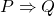Many of the statements we want to prove in mathematics are conditionals. How could we go about doing this? Actually it's easy. Recall the truth table for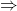: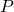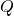T T T 1 F F 2 F T T 3 F T 4

We want to establish thatis true. That is, we want to make sure that we end up with a T in the third column of the truth table above. Phrased this way, it looks like our job is pretty easy (because there are so many Ts in that column!). Ifis false, we're good to go. So we may as well assumeis true. Then we only have to worry aboutbeing false. Sinceis a statement, it's either true or false. So ruling outbeing false means showingis true.

Thus: we need to assumeis true and proveis true. The shorthand for this is: assume; prove. In fact many primers on proof will belabor this point, putting the above maxim in a box in some bold color and telling you that now you know how to prove conditionals! But of course it's how one gets from the assumption ofto the conclusion ofthat is where the fun lies, and we haven't said really anything about that (yet). This is called a direct proof of.

### other uses of the truth table for#### modus ponens

Now let's imagine that we knowis true; in other words, that we're in line 1, line 3, or line 4; but we don't know which one. If we had some additional information, like we happened to know thatwas true, then we'd know for sure we're in line 1. But then we'd also know thatis true.

So we have the following rule: if we knowand we know, then we knowalso.

If you think about it for a moment, this is more or less the definition of the word if. But Classical and medieval logicians gave it a name, modus ponens.

#### modus tollens

What if we happened to know thatwas false? Then we've got to be in line 4, so we know thatis also false. So we have the following rule:

If we knowis false and we know, then we knowis false also.

This is known to the medieval logicians as modus tollens.

If modus tollens seems a bit odd to you, just consider the following statement:

When you see ice in my scotch, that'll be the day I die!

How does this work? Logically, the speaker is asserting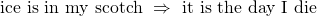. But since they're around to have the conversation with us, we know the consequent is false. So the intended meaning must be that the antecedent was false, i.e. there's no ice in their scotch. In fact we use this sort of discourse all the time in ordinary conversation.

A similar mode of reasoning also relies on the fact that only a false statement can imply a false statement. The idea is to establish a claim (we'll call it) by showing thatcouldn't possibly be not true. So we assume, and make valid logical steps until we get to some egregious statementwhich we all agree is false. Since our reasoning was sound, this must have meant thatwas false, i.e. thatwas true. for example:

Consider what would happen if lowering the income tax rate always lowered income tax revenues. If we set the income tax rate at 100\%, we'd bring in more income tax revenue than any other rate. But at a 100\% income tax rate, nobody will have any reason to work, hence there will be no income tax revenue at all. Thus we should expect to get no income tax revenue at any other rate. On the other hand, the current income tax rate does generate income tax revenue. So we see that sometimes, lowering the income tax rate may increase income tax revenues.

Exercise. In the above argument, identify:, the goal we set to prove;, the thing we assumed;, the truly egregious statement we all agree is false.

"modus ponens" is often translated as "the method of affirming", but that's a bad translation. In Latin, "pono" means "to put" or "to place". So you might call this "the placement method". Why? If you've ever watched a legal drama, the lawyers say things like "I put it to you, the jury, that...". That's sot of what's going on here.

"modus tollens" is often translated as "the method of denying", but again that's bad Latin. "tollo" means "to snatch". My preferred translation of this would be something like "the thief's method".

Actually both modus ponens and moduls tollens are less mysterious than they are made to seem by their fancy names. Perhaps the best name for both would be "modus manifestissumus" -- "the really obvious method".

"reductio ad absurdum" actually gets translated correctly, as "reduction to the absurd". The idea is to take the denial of what you want to prove, and turn it into something. . . absurd.

### some other useful logical facts

Below is a list of logical facts. You should establish each one by using one or more of the following ways: making a truth table for the formulae involved; comparing truth tables; making algebraic manipulations of the formulae. You should also convince yourself that the statements make sense without resorting to these formal, abstract methods.

1. If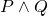is true, thenis true.
2. Ifis true, then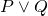is true.
3. Ifand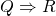are both true, then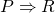is true.
4.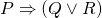is equivalent to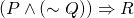and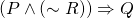.
5.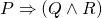is equivalent to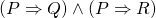.
6. Ifis true andis true, then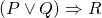is true.
7. Ifis true, thenis true andis true.

We can restate each of the above facts as the claim that a certain logical formula is a tautology:

1.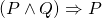is a tautology.
2.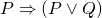is a tautology.
3.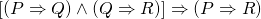is a tautology.
4.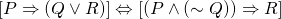is a tautology.
5.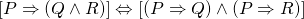is a tautology.
6.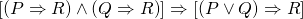is a tautology.
7.is a tautology.